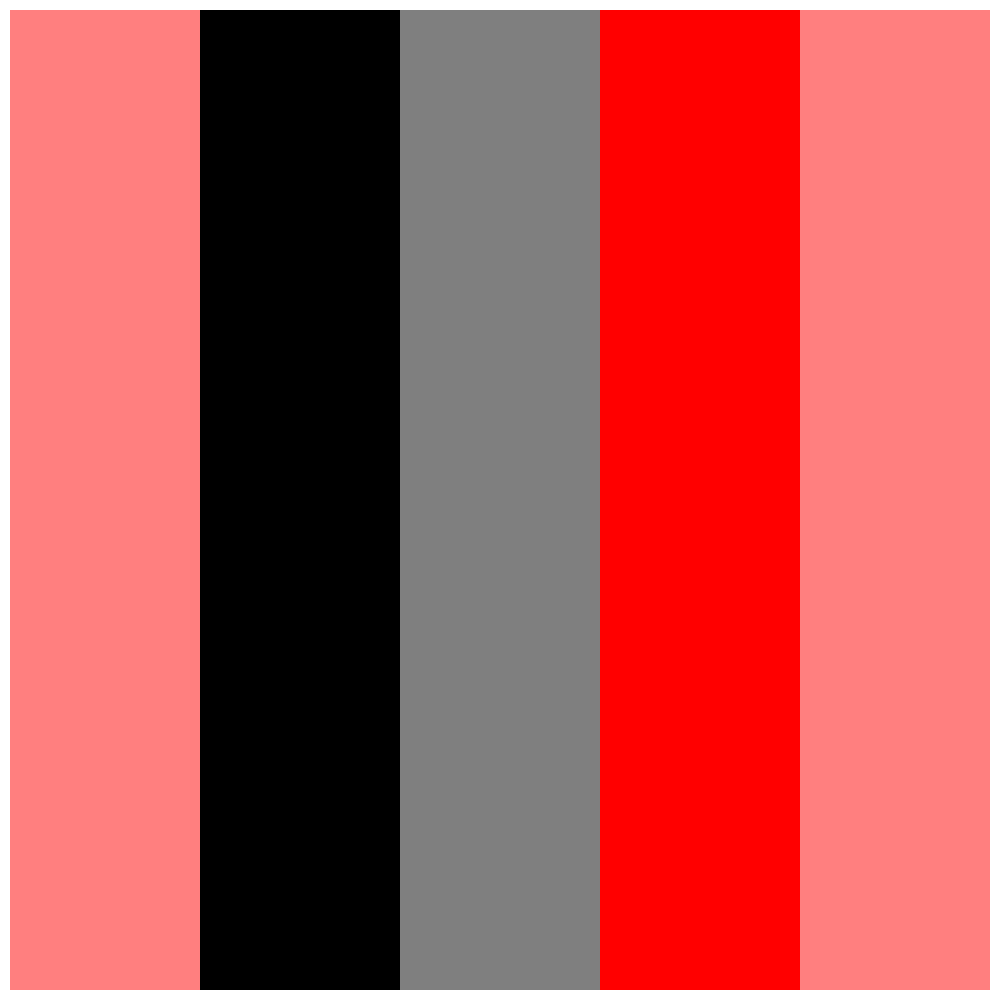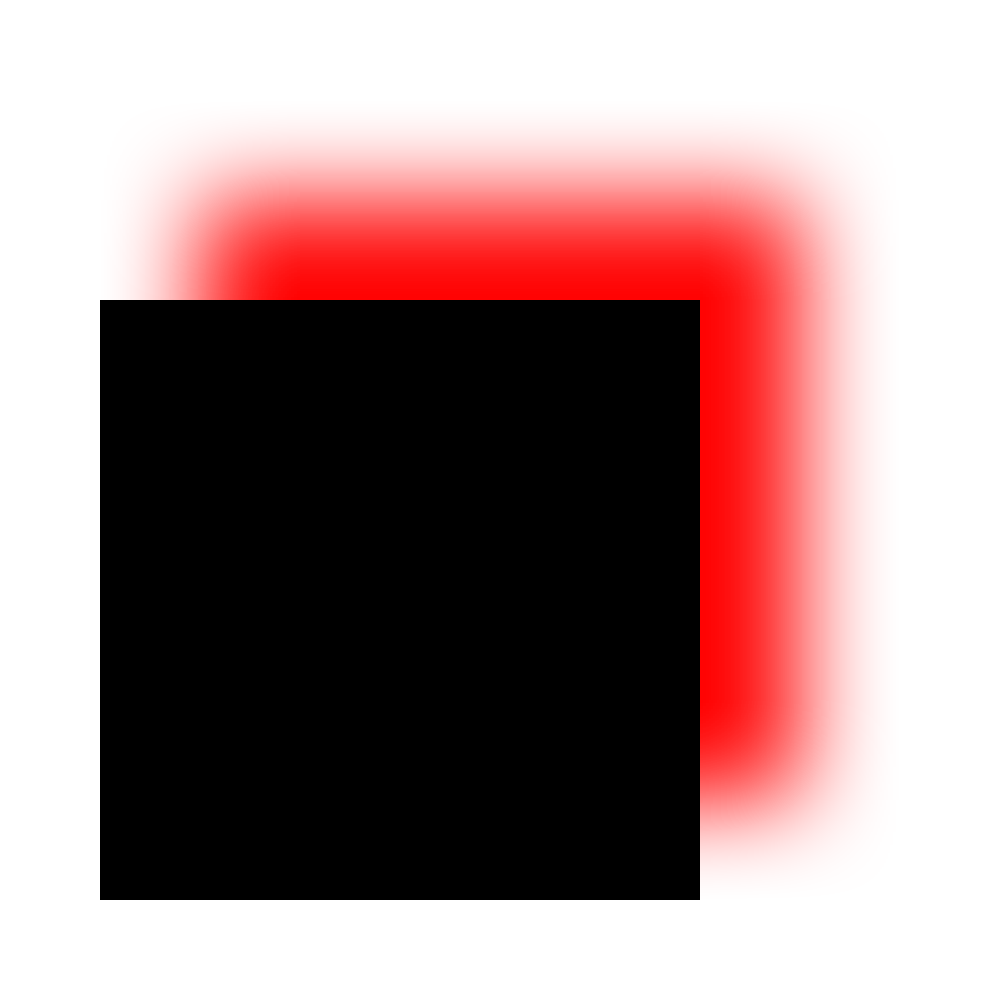# Fill¶

Set a fill before drawing a shape.

`fill`(r=None, g=None, b=None, alpha=1)

Sets the fill color with a red, green, blue and alpha value. Each argument must a value float between 0 and 1.

```fill(1, 0, 0, .5)
# draw a rect
rect(10, 10, 200, 980)

# only set a gray value
fill(0)
# draw a rect
rect(200, 10, 200, 980)

# only set a gray value with an alpha
fill(0, .5)
# draw a rect
rect(400, 10, 200, 980)

# set rgb with no alpha
fill(1, 0, 0)
# draw a rect
rect(600, 10, 200, 980)

# set rgb with an alpha value
fill(1, 0, 0, .5)
# draw a rect
rect(800, 10, 190, 980)
````linearGradient`(startPoint=None, endPoint=None, colors=None, locations=None)

• startPoint as (x, y)
• endPoint as (x, y)
• colors as a list of colors, described similary as fill
• locations of each color as a list of floats. (optionally)

Setting a gradient will ignore the fill.

```# set a gradient as the fill color
(100, 100),                         # startPoint
(800, 800),                         # endPoint
[(1, 0, 0), (0, 0, 1), (0, 1, 0)],  # colors
[0, .2, 1]                          # locations
)
# draw a rectangle
rect(10, 10, 980, 980)
````radialGradient`(startPoint=None, endPoint=None, colors=None, locations=None, startRadius=0, endRadius=100)

• startPoint as (x, y)
• endPoint as (x, y)
• colors as a list of colors, described similary as fill
• locations of each color as a list of floats. (optionally)

Setting a gradient will ignore the fill.

```# set a gradient as the fill color
(300, 300),                         # startPoint
(600, 600),                         # endPoint
[(1, 0, 0), (0, 0, 1), (0, 1, 0)],  # colors
[0, .2, 1],                         # locations
)
# draw a rectangle
rect(10, 10, 980, 980)
````shadow`(offset, blur=None, color=None)

Adds a shadow with an offset (x, y), blur and a color. The color argument must be a tuple similarly as fill. The offset`and `blur argument will be drawn independent of the current context transformations.

```# a red shadow with some blur and a offset
shadow((100, 100), 100, (1, 0, 0))
# draw a rect
rect(100, 100, 600, 600)
```